# DSE 6000 Final Project Analysis - Wayne State University¶

By Domeda Duncan, Robert Gastineau, Randeep Singh, Devon Ankar (Contact: devon@devonankar.com)

We chose the following three job portals to scrape data from:

• Monster
• ZipRecruiter
• SimplyHired

In :
#imports
import pandas as pd
import numpy as np
from collections import Counter
%matplotlib inline
import matplotlib.pyplot as plt
import seaborn as sns

#pull CSVs into dataframes

#main CSVs
monster = pd.read_csv('data\CleanedMonster.csv', sep='|', index_col=False, encoding='latin1')
ziprecruiter = pd.read_csv('data\cleanedziprec.csv', sep='|', index_col=False, encoding='latin1')
simplyhired = pd.read_csv('data\CleanedSimply.csv', sep='|', index_col=False, encoding='latin1')

#skills CSVs
monsterskills = pd.read_csv('data\WordCount_Monster.csv', sep='|', index_col=False, encoding='latin1')
ziprecruiterskills = pd.read_csv('data\WordCount_Zip.csv', sep='|', index_col=False, encoding='latin1')
simplyhiredskills = pd.read_csv('data\WordCount_Simply.csv', sep='|', index_col=False, encoding='latin1')

monster.shape + ziprecruiter.shape + simplyhired.shape

Out:
(760, 8, 342, 8, 1330, 10)
In :
#append dataframes into one dataframe
df1 = monster.append(ziprecruiter, ignore_index=True)
alldata = df1.append(simplyhired, ignore_index=True)

alldata.shape

Out:
(2432, 10)
• Monster: 760 jobs
• ZipRecruiter: 342 jobs
• SimplyHired: 1330 jobs

Total: 2432 jobs

In :
#append dataframes into one dataframe
df2 = monsterskills.append(ziprecruiterskills, ignore_index=True)
allskills = df2.append(simplyhiredskills, ignore_index=True)


Problems discovered later that we want to correct at the beginning:

• Naming convention variations, e.g. "New York City" vs. "New York" (in the city field) refer to the same place
• Solution: replace New York City with New York, South San Francisco with San Francisco, etc.
• Some entries use full state name instead of two-letter state code, e.g. "Michigan" instead of "MI"
• Solution: get reference table of state code and state name, then replace state name with state code
• Within these, some full state names have the first letter cut off, e.g. "irginia" for VA
• Other issues with the state field as corrected below
In :
#city name replacements
alldata['City'] = alldata['City'].replace(['New York City','new york city','NEW YORK CITY'], 'New York')
alldata['City'] = alldata['City'].replace('South San Francisco', 'San Francisco')

#import state code reference table

#replace any full state names with state codes
alldata['State'] = alldata.State.replace(statecodes.set_index('State').Abbreviation.to_dict())

#replace any cut-off state names with state codes
alldata['State'] = alldata.State.replace(statecodes.set_index('StatewoFirst').Abbreviation.to_dict())

#other issues with state field
alldata['State'] = alldata['State'].replace('ome Based', 'Home Based')
alldata['State'] = alldata['State'].replace('emote', 'Remote')
alldata['State'] = alldata['State'].replace('one', 'None')
alldata['State'] = alldata['State'].replace('nited States', 'United States')
alldata['State'] = alldata['State'].replace('AZ, United States, AZ', 'AZ')


## 1. What are the typical job titles listed in Data Science?¶

### Since our search string in all three job portals was "Data Scientist", we hypothesize that the most common job title will be Data Scientist.¶

Assumptions:

• Regarding typos and other entry errors: we noticed a few job titles that were misspelled, included the company name, included the city name (e.g. "Data Scientist - Seattle, WA"), or included abbreviations (e.g. "Sr. Data Scientist"). Such variations are inevitable in large datasets, but we assume they are not frequent enough to change the overall result found below.
In :
titlecounter = Counter(alldata['Title'])
len(titlecounter.keys())

Out:
1450

There are 1450 unique job titles among 2432 jobs.

In :
titlecount2 = pd.DataFrame([titlecounter])
titlecount = titlecount2.transpose()

plt.ticklabel_format(style='plain', axis='x')
titlecountplot=titlecount.nlargest(20,0)
sns.barplot(y=titlecountplot.index, x=titlecountplot)
plt.title('20 most common job titles within Data Science')
plt.ylabel('Job Titles')
plt.xlabel('Frequency/Count')

Out:
Text(0.5,0,'Frequency/Count')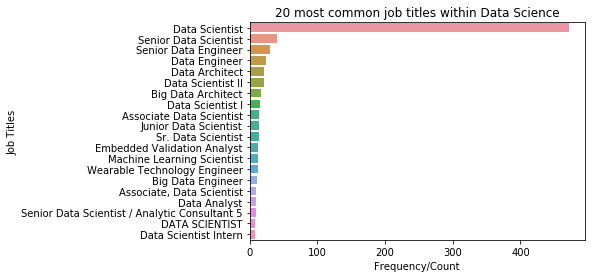In :
titlecountplot.head(10)

Out:
0
Data Scientist 471
Senior Data Scientist 41
Senior Data Engineer 30
Data Engineer 24
Data Architect 21
Data Scientist II 21
Big Data Architect 16
Data Scientist I 15
Associate Data Scientist 14
Junior Data Scientist 14

## 2. Where are data scientist jobs located the most? Geographical distribution of jobs in this segment? (State and City levels)¶

### State-level: there will likely be a lot of data scientist jobs in California.¶

In :
statecounter = Counter(alldata['State'])
len(statecounter.keys())

Out:
55

There are 55 unique states represented!

Possibilities:

• "United States" incorrectly listed under the state field
• Job has multiple locations: multiple states are listed in the state field
• Jobs with "None", remote jobs, home-based jobs
• For international jobs, there is no field for the country, so international companies or recruiters may put their country in the state field

Assumptions:

• We again assume that the above errors are a negligible percentage of total job entries and do not change the overall trends
In :
statecount2 = pd.DataFrame([statecounter])
statecount = statecount2.transpose()

plt.ticklabel_format(style='plain', axis='x')
statecountplot=statecount.nlargest(25,0)
sns.barplot(y=statecountplot.index, x=statecountplot)
plt.title('Geographic distribution of data science jobs')
plt.ylabel('States')
plt.xlabel('Frequency/Count')

Out:
Text(0.5,0,'Frequency/Count')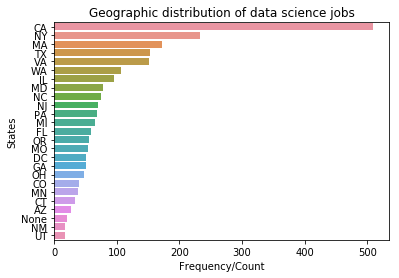In :
statecountplot.head(5)

Out:
0
CA 509
NY 233
MA 172
TX 153
VA 151

### City-level: we hypothesize that data scientist jobs will be located primarily around urban centers and high population areas, i.e. major cities.¶

Issues:

• Similar or nearby cities are differentiated, e.g. "Dearborn" vs. "Detroit"
• This information is extremely valuable to individual job seekers, but dilutes the high-level geographic picture we are seeking
In :
#add a city-state field to alldata
alldatawithcitystate = alldata.assign(city_state = alldata['City'] + "-" + alldata['State'])

citycounter = Counter(alldatawithcitystate['city_state'])

citycount2 = pd.DataFrame([citycounter])
citycount = citycount2.transpose()

plt.ticklabel_format(style='plain', axis='x')
citycountplot=citycount.nlargest(20,0)
sns.barplot(y=citycountplot.index, x=citycountplot)
plt.title('Geographic distribution of data science jobs')
plt.ylabel('Cities')
plt.xlabel('Frequency/Count')

Out:
Text(0.5,0,'Frequency/Count')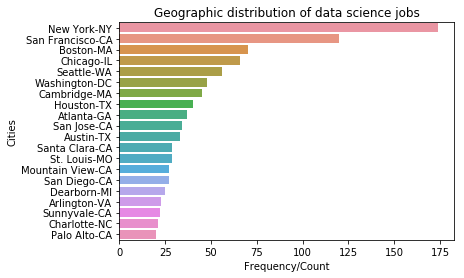### Visualize geographic distribution¶

Cross-reference publicly available table of latitudes and longitudes

In :
#import coordinates csv file

#remove duplicates from coordinates
coordinates = coordinates.drop_duplicates(subset=['city','state','county'], keep='first')

#add a city-state field to coordinates
coordinates = coordinates.assign(city_state = coordinates['city'] + "-" + coordinates['state'])

citycount = citycount.assign(city_state = citycount.index)
citycount = citycount.merge(coordinates, left_on='city_state', right_on='city_state', how='outer')

In :
x2 = citycount['longitude']
y2 = citycount['latitude']
area = citycount
colors = 'b'
alpha2 = 0.7

plt.scatter(x2, y2, s=area, c=colors, marker='o', alpha=alpha2, linewidths=1, edgecolors='face')
plt.title('Geographic distribution of data science jobs by coordinates')
plt.xlabel('longitude')
plt.ylabel('latitude')

plt.show()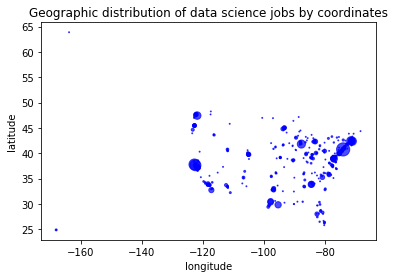Map of the US! Complete with small spots for Alaska (top left corner) and Hawaii (bottom left corner). We see again that jobs are concentrated in the Pacific Northwest, California, and the East Coast (NY, MA, VA, DC).

## 3. What skills are in high demand with employers in this segment? What combinations of skills appear most often together?¶

### We hypothesize that technologies we learned in this class are in higher demand than older technologies (e.g. SQL) or technologies that are used for smaller datasets (e.g. Excel). In addition, we hypothesize that statistical packages such as R/RStudio, SAS, and SPSS are also in high demand.¶

Assumptions:

• Could not look for "R" because it is a letter; tried " R ", " R,", etc. but it still searched for the letter "R" which will obviously appear many times outside of the context we need.
• Need to exclude skill "SED" because "SED" appears within a lot of other words and that caused a bunch of false positives.
In :
skillcounter = Counter(allskills['Words'])
len(skillcounter.keys())

skillcount2 = pd.DataFrame([skillcounter])
skillcount = skillcount2.transpose()

#exclude skill "SED" because "SED" appears within a lot of other words and that caused a bunch of false positives.
skillcount = skillcount[skillcount.index != 'SED']

plt.ticklabel_format(style='plain', axis='x')
skillcountplot=skillcount.nlargest(25,0)
sns.barplot(y=skillcountplot.index, x=skillcountplot)
plt.title('25 most in-demand skills within Data Science')
plt.ylabel('Skills')
plt.xlabel('Frequency/Count')

Out:
Text(0.5,0,'Frequency/Count')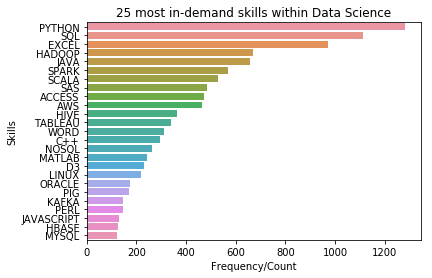In :
skillcountplot.head(10)

Out:
0
PYTHON 1283
SQL 1112
EXCEL 970
JAVA 656
SPARK 568
SCALA 530
SAS 486
ACCESS 473
AWS 463

### The top 5 skills are PYTHON, SQL, EXCEL, Hadoop, and Java. Our hypothesis is only partially correct.¶

In this class, we learned Python and Hadoop, but we did not learn SQL, Java, or Excel. It is only partially true that technologies we learned in this class are in higher demand than older technologies or technologies useful for smaller datasets. Also, statistical packages did not make the top 10 skills except for SAS at no. 9.

### What combinations of skills appear most often together?¶

In :
df4=allskills[allskills['Words']!='SED']

df6=df4[['key','Words']]
df6.index=df6['key']
df6Dup = df6

# Now to do a cross join to itself
df7 = df6.join(df6Dup, lsuffix='_1', rsuffix='_2')

# Now we want to drop the records where Words_1=Words_2 and Words 1 < Words_2
df8=df7[df7['Words_1']<df7['Words_2']]

df8= df8.reset_index(drop=True)
df8['freqCombo']=df8.groupby(['Words_1','Words_2'])['Words_1'].transform('count')

df9 = df8[['Words_1','Words_2','freqCombo']]
df9 = df9.sort_values('freqCombo', ascending=False)
ComboFreq=df9.drop_duplicates(subset=['Words_1','Words_2'])

      Words_1 Words_2  freqCombo
59897  PYTHON     SQL       1495
67948   EXCEL  PYTHON       1124
56908   EXCEL     SQL       1072
36395    JAVA  PYTHON        994
7694   PYTHON   SPARK        859
24317    JAVA     SQL        820
61866   SPARK     SQL        733
6421   PYTHON   SCALA        726
18724     AWS  PYTHON        649
30189   SCALA     SQL        623
33521   EXCEL    JAVA        617
21707     AWS     SQL        593
25963  PYTHON     SAS        564
76062     SAS     SQL        552
43181  ACCESS  PYTHON        550


The above are the top 20 combinations of skills that occur most often together.

## 4. Salary range and its correlation to location if any.¶

### We hypothesize that higher cost-of-living areas on the coasts and in urban centers/cities have higher salaries than inland or rural areas.¶

Assumptions:

• Only SimplyHired provides salary information; the other two job portals do not
• We assume that salaries on SimplyHired are representative of overall salaries
• Even within SimplyHired, some jobs were missing salary information: they show salary of 0 or blank
• Again, we assume that the salaries we do have are representative of those we don't
In :
#create new dataframe with just the salary information
salaryfilter1 = alldata.filter(items=['Title','City', 'State', 'Min_Salary', 'Max_Salary'])

#filter out the nan and 0s
salaryfilter2 = salaryfilter1[salaryfilter1['Min_Salary'].notnull() & (salaryfilter1['Max_Salary'].notnull())
& (salaryfilter1['Min_Salary'] !=0) & (salaryfilter1['Max_Salary'] != 0)]
#& (salaryfilter1['Title'] == 'Data Scientist')

#let's see what the smallest salaries are
#salaryfilter2.sort_values(['Min_Salary'], ascending=True)


Salaries of just 2000/year are almost certainly incorrect. Let's filter out salaries under 12000/year (since that's 1000/month). Generally, Data Scientist positions requiring MS will pay much more than that.

In :
salaryfilter2 = salaryfilter2[salaryfilter2['Min_Salary'] >= 12000]
#salaryfilter2.sort_values(['Min_Salary'], ascending=True)

In :
#check the high end too
#salaryfilter2.sort_values(['Max_Salary'], ascending=False)
#highest Max_Salary is 300,000/year, which is probably correct

In :
#min values for both min and max salary range
print(salaryfilter2['Min_Salary'].min(), "-", salaryfilter2['Max_Salary'].min())

12000.0 - 15000.0

In :
#max values for both min and max salary range
print(salaryfilter2['Min_Salary'].max(), "-", salaryfilter2['Max_Salary'].max())

200000.0 - 300000.0

In :
#filter on title = data scientist
salaryfilter3 = salaryfilter2[salaryfilter2['Title'] == 'Data Scientist']

#min values for both min and max salary range - for data scientist title only
print(salaryfilter3['Min_Salary'].min(), "-", salaryfilter3['Max_Salary'].min())

20000.0 - 45000.0

In :
#max values for both min and max salary range - for data scientist title only
print(salaryfilter3['Min_Salary'].max(), "-", salaryfilter3['Max_Salary'].max())

190000.0 - 230000.0


For all job titles: The lowest salary in the dataset is 12,000 - 15,000 per year. The highest salary in the dataset is 200,000 - 300,000 per year.

For data scientists: The lowest salary in the dataset is 20,000 - 45,000 per year. The highest salary in the dataset is 190,000 - 230,000 per year.

Next, test hypothesis by checking for correlation of salary to location (if any).

In :
salaryfilter4 = salaryfilter2.filter(items=['City', 'State', 'Max_Salary'])
salaryfilter4 = salaryfilter4.assign(city_state = salaryfilter4['City'] + "-" + salaryfilter4['State'])
avgmaxsalaries = salaryfilter4.groupby('city_state', as_index=False).Max_Salary.mean().round(2)

plt.ticklabel_format(style='plain', axis='x')
salaryplot = avgmaxsalaries.nlargest(20,'Max_Salary')
sns.barplot(y=salaryplot['city_state'], x=salaryplot['Max_Salary'])
plt.title('Top 20 cities by salary')
plt.ylabel('Cities')
plt.xlabel('Average Max Salary')

Out:
Text(0.5,0,'Average Max Salary')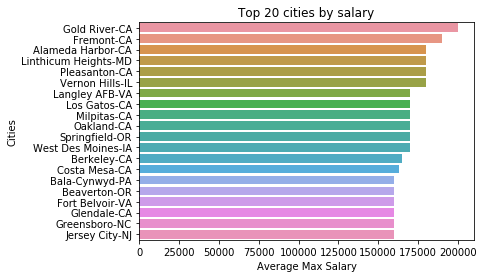### Locations in CA, MD, IL, VA, OR, and other higher cost-of-living areas have higher salaries on average.¶

In :
avgmaxsalaries = avgmaxsalaries.merge(coordinates, left_on='city_state', right_on='city_state', how='outer')

In :
x2 = avgmaxsalaries['longitude']
y2 = avgmaxsalaries['latitude']
area = avgmaxsalaries['Max_Salary']/4000 #without this factor, the numbers are too large and it overwhelms the map!
colors = 'g'
alpha2 = 0.5

plt.scatter(x2, y2, s=area, c=colors, marker='x', alpha=alpha2, linewidths=1, edgecolors='face')
plt.title('Geographic distribution of salaries by coordinates')
plt.xlabel('longitude')
plt.ylabel('latitude')

plt.show()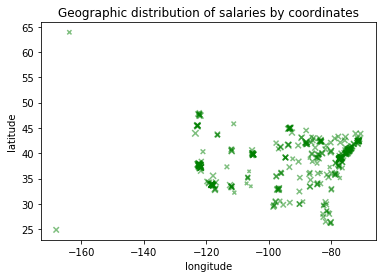### We can see that salaries are larger on the coasts and in urban areas. CA, PNW, East Coast, Chicago, etc.¶

Note: We are using average salary for each city, so the number of jobs in each city (number of observations) does not weight the size of each bubble. For example, San Francisco's bubble is not bigger because there are more jobs there; it's bigger because the average salary is higher there.

However, without significance testing, we don't know if these differences in salaries are statistically significant.